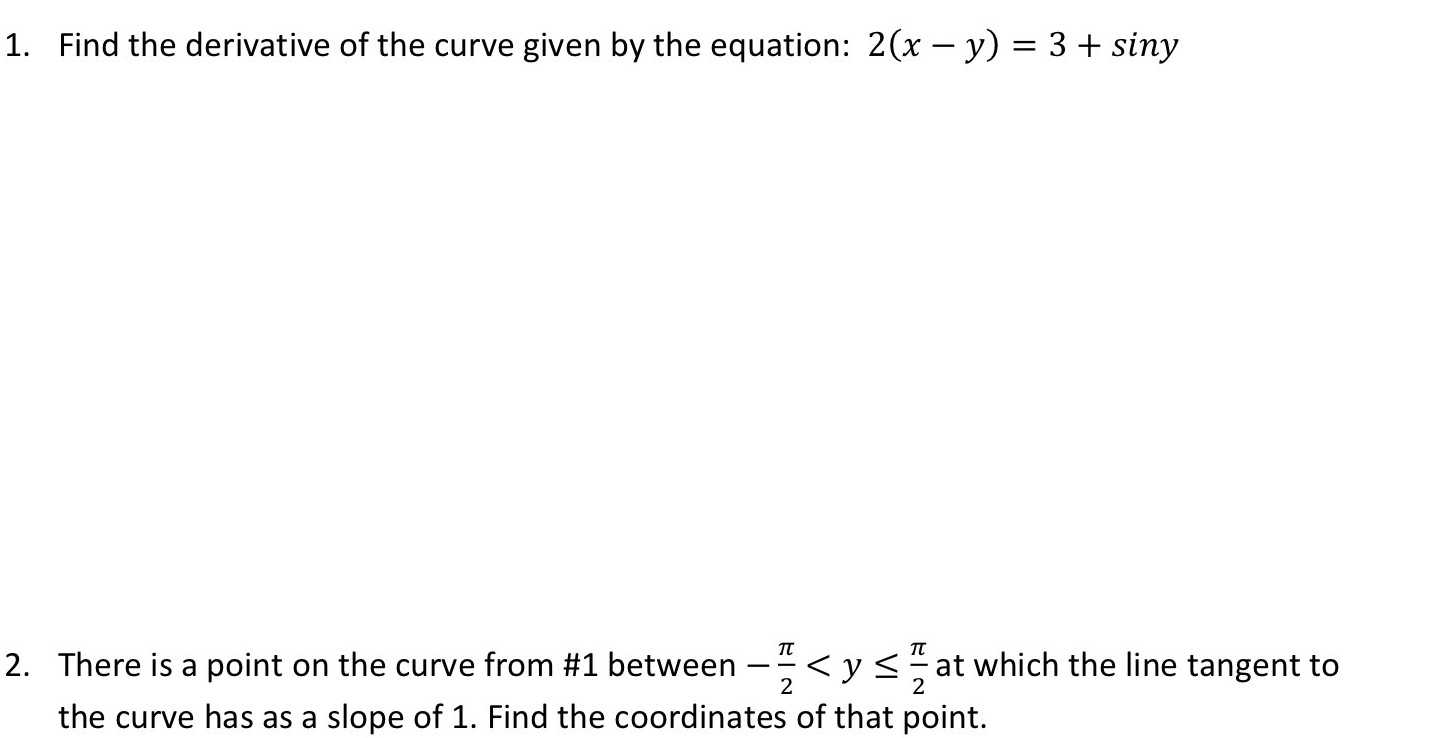### ¿Todavía tienes preguntas de matemáticas?

Pregunte a nuestros tutores expertos
Algebra
Pregunta1. Find the derivative of the curve given by the equation: $$2 ( x - y ) = 3 + \sin y$$

2. There is a point on the curve from #1 between $$- \frac { \pi } { 2 } < y \leq \frac { \pi } { 2 }$$ at which the line tangent to the curve has as a slope of 1. Find the coordinates of that point.

$$\frac{1}{2}\cos y+ 1\\(2+ \frac{\pi}{2},0 )$$